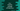# C++ program to find the average of three numbers## C++ program to find the average of three numbers:

In this post, we will learn how to find the average of three numbers in C++. Our program will take the numbers as inputs from the user and print the average of these numbers.

We will solve it in two different ways: without using any function and by using a function.

You will learn how to take user-inputs, how to do mathematical calculations and how to print result in C++.

### Algorithm to use:

We need to find the average of three numbers. The average of three numbers is:

``sum/3``

So, the program will have to follow the following steps to find the average:

• Take the numbers as inputs from the user
• Find the sum of these numbers
• Divide the sum by 3 to find the average
• Print the average

### C++ program to find the average of three numbers without using a function:

Let’s try it in a simple way first. We will write a program that will take the numbers as inputs and print the average of these numbers.

``````#include <iostream>

using namespace std;

int main()
{
float first, second, third, sum, avg;

cout << "Enter the first number: " << endl;
cin >> first;

cout << "Enter the second number: " << endl;
cin >> second;

cout << "Enter the third number: " << endl;
cin >> third;

sum = first + second + third;
avg = sum / 3;

cout << "Average value: " << avg << endl;

return 0;
}``````

Here,

• first, second and third are three floating point variables to hold the numbers.
• sum is to hold the sum of these variables and avg is to hold the average value.
• By using cout and cin, it asks the user to enter the numbers, reads them and stores them in the variables we defined above.
• The sum of these numbers is calculated and stored in sum.
• The average is calculated by dividing the sum by 3. This value is stored in avg.
• The last line is printing the average value it calculated.

If you run this program, it will print output as like below:

``````Enter the first number:
12.5
Enter the second number:
22.45
Enter the third number:
55.67
Average value: 30.2067

Enter the first number:
10
Enter the second number:
20
Enter the third number:
30
Average value: 20``````

### C++ program to find the average of three numbers by using a separate function:

We can also use a separate function to find the average of three numbers. The main program will call this function, it will return the final result and the main program will print the result.

Let’s take a look at the program that uses a function:

``````#include <iostream>

using namespace std;

float findAverage(float x, float y, float z)
{
return (x + y + z) / 3;
}

int main()
{
float first, second, third;

cout << "Enter the first number: " << endl;
cin >> first;

cout << "Enter the second number: " << endl;
cin >> second;

cout << "Enter the third number: " << endl;
cin >> third;

cout << "Average value: " << findAverage(first, second, third) << endl;

return 0;
}``````

This is almost similar to the above program. We are taking the numbers as inputs and storing them in first, second and third float variables.

But, we have created a different method to find the average. It is findAverage. This method takes the numbers as arguments and returns the average value.

If you run this program, it will give similar output:

``````Enter the first number:
12
Enter the second number:
13
Enter the third number:
14
Average value: 13``````

The advantage of using a separate function or method is that you can call it from anywhere you want. If you have a lot of classes in your program, you can call the exact same method from all of these files. If you want to make change to the function, you can do it at one place i.e. inside the function and it will reflact on all other places.

Let me show you how to use a class to do it.

### C++ program to find the average value of three numbers by using a class:

We can also use a separate class to find the average. For example:

``````#include <iostream>

using namespace std;

class Calculator
{
public:
float findAverage(float x, float y, float z)
{
return (x + y + z) / 3;
}
};

int main()
{
float first, second, third;
Calculator c;

cout << "Enter the first number: " << endl;
cin >> first;

cout << "Enter the second number: " << endl;
cin >> second;

cout << "Enter the third number: " << endl;
cin >> third;

cout << "Average value: " << c.findAverage(first, second, third) << endl;

return 0;
}``````

This program uses a separate class Calculator that has one method findAverage to find the average of three numbers.

We are calling this method of this class from the main method.

It will give similar output.

You can also move everything to a class method. The class method will read the values, find the average and print the result.

``````#include <iostream>

using namespace std;

class Calculator
{
public:
void findAverage()
{
float first, second, third;
cout << "Enter the first number: " << endl;
cin >> first;

cout << "Enter the second number: " << endl;
cin >> second;

cout << "Enter the third number: " << endl;
cin >> third;
cout << "Average value: " << (first + second + third) / 3 << endl;
}
};

int main()
{

Calculator c;
c.findAverage();

return 0;
}``````

So, I moved the code inside the findAverage method. Inside main, I am creating an object of the Calculator class and calling this method using that object.

It will give similar results.